Problem

# The 0.1-lb golf ball is struck by the club and then travels along the trajectory shown. De...

The 0.1-lb golf ball is struck by the club and then travels along the trajectory shown. Determine the average impulsive force the club imparts on the ball if the club maintains contact with the ball for 0.5 ms.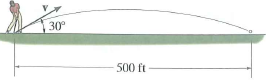#### Step-by-Step Solution

Solution 1

Weight of the golf ball is $$W=0.1 \mathrm{lb}$$

Position of the ball along the $$x$$ -motion is expressed as

$$s_{x}=s_{0}+\left(v_{0}\right)_{x} t$$

Where, $$\left(v_{0}\right)_{x}=v \cos 30^{\circ}$$

$$\left(v_{0}\right)_{x}=0.866 v$$

We have $$s_{x}=500 \mathrm{ft}$$ and $$s_{0}=0$$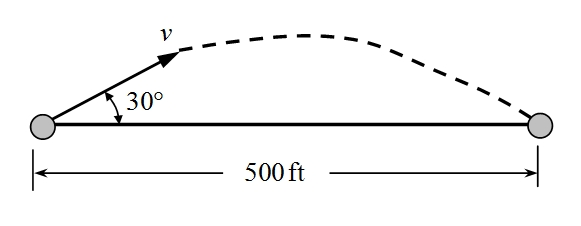Accordingly, we have from (1)

$$500=0+0.866 v \times t$$

$$t=\frac{500}{0.866 v}$$

$$t=\frac{577.367}{v} \quad \ldots \ldots . .$$

We have vertical motion of the golf ball as

$$s_{y}=\left(s_{0}\right)_{y}+\left(v_{0}\right)_{y} t+\frac{1}{2} a_{y} t^{2}$$

Where, we have $$s_{y}=0 ;\left(s_{0}\right)_{y}=0 ; a_{y}=-g$$

By substituting the values of the parameters into (3), we have

$$0=0+v \sin 30^{\circ} \times\left(\frac{577.367}{v}\right)+\frac{1}{2} \times(-32.2) \times\left(\frac{577.367}{v}\right)^{2}$$

$$0=288.6835-\frac{5366977.71}{v^{2}}$$

$$v^{2}=\frac{5366977.71}{288.6835}$$

$$v=136.35 \mathrm{ft} / \mathrm{s}$$

From the principle of linear impulse and momentum, we have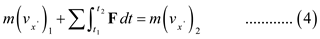Initial speed of the golf ball is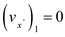Speed of the golf ball, is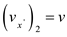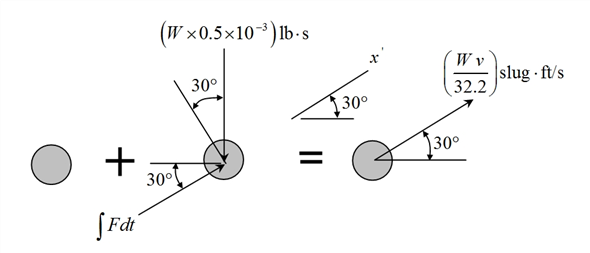By substituting the values of the parameters into $$(4)$$, we have

$$\left(\frac{0.1}{32.2}\right) \times 0+F_{\text {avg }} \times(0.0005)=\left(\frac{0.1}{32.2}\right) \times 136.35$$

$$0.0005 F_{\text {ang }}=0.42345 \mathrm{lb}$$

$$F_{a y g}=846.9 \mathrm{lb}$$

Average impulsive force the club imparts on the ball is $$\quad F_{\text {avg }}=846.9 \mathrm{lb}$$

Add your Solution
Textbook Solutions and Answers Search
Solutions For Problems in Chapter 15